JEE  >  Test: Vectors- Assertion & Reason Type Questions

# Test: Vectors- Assertion & Reason Type Questions - JEE

Test Description

## 6 Questions MCQ Test Mathematics (Maths) Class 12 - Test: Vectors- Assertion & Reason Type Questions

Test: Vectors- Assertion & Reason Type Questions for JEE 2023 is part of Mathematics (Maths) Class 12 preparation. The Test: Vectors- Assertion & Reason Type Questions questions and answers have been prepared according to the JEE exam syllabus.The Test: Vectors- Assertion & Reason Type Questions MCQs are made for JEE 2023 Exam. Find important definitions, questions, notes, meanings, examples, exercises, MCQs and online tests for Test: Vectors- Assertion & Reason Type Questions below.
Solutions of Test: Vectors- Assertion & Reason Type Questions questions in English are available as part of our Mathematics (Maths) Class 12 for JEE & Test: Vectors- Assertion & Reason Type Questions solutions in Hindi for Mathematics (Maths) Class 12 course. Download more important topics, notes, lectures and mock test series for JEE Exam by signing up for free. Attempt Test: Vectors- Assertion & Reason Type Questions | 6 questions in 12 minutes | Mock test for JEE preparation | Free important questions MCQ to study Mathematics (Maths) Class 12 for JEE Exam | Download free PDF with solutions
 1 Crore+ students have signed up on EduRev. Have you?
Test: Vectors- Assertion & Reason Type Questions - Question 1

### Direction: In the following questions, A statement of Assertion (A) is followed by a statement of Reason (R). Mark the correct choice asAssertion (A): The position vector of a point say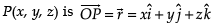and its magnitude is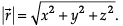Reason (R): If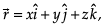then coefficient of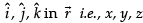are called the direction ratios of vector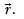Detailed Solution for Test: Vectors- Assertion & Reason Type Questions - Question 1
Assertion (A) and Reason (R) both are individually correct.
Test: Vectors- Assertion & Reason Type Questions - Question 2

### Direction: In the following questions, A statement of Assertion (A) is followed by a statement of Reason (R). Mark the correct choice asAssertion (A): The area of parallelogram with diagonals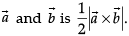Reason (R): If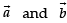represent the adjacent sides of a triangle, then the area of triangle can be obtained by evaluating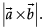Detailed Solution for Test: Vectors- Assertion & Reason Type Questions - Question 2
If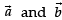represent the adjacent sides of a triangle, then the area of triangle can be obtained by evaluating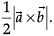Test: Vectors- Assertion & Reason Type Questions - Question 3

### Direction: In the following questions, A statement of Assertion (A) is followed by a statement of Reason (R). Mark the correct choice asAssertion (A): The direction of cosines of vector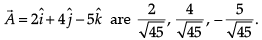Reason (R): A vector having zero magnitude and arbitrary direction is called ‘zero vector’ or ‘null vector’.

Detailed Solution for Test: Vectors- Assertion & Reason Type Questions - Question 3
Assertion (A) is correct.

Direction cosines of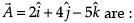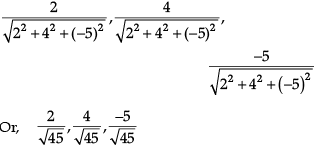Test: Vectors- Assertion & Reason Type Questions - Question 4

Direction: In the following questions, A statement of Assertion (A) is followed by a statement of Reason (R). Mark the correct choice as

Assertion (A): The position of a particle in a rectangular coordinate system is (3, 2, 5). Then its position vector be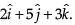Reason (R): The displacement vector of the particle that moves from point P(2, 3, 5) to point Q(3, 4, 5) is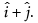Detailed Solution for Test: Vectors- Assertion & Reason Type Questions - Question 4
Assertion (A) is wrong.

The position of a particle in a rectangular coordinate system is (3, 2, 5). Then its position vector be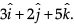Reason (R) is correct.

The displacement vector of the particle that moves from point P(2, 3, 5) to point Q(3, 4, 5)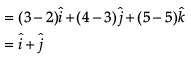Test: Vectors- Assertion & Reason Type Questions - Question 5

Direction: In the following questions, A statement of Assertion (A) is followed by a statement of Reason (R). Mark the correct choice as

Assertion (A): The vectors which can undergo parallel displacement without changing its magnitude and direction are called free vectors.

Reason (R):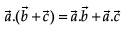Detailed Solution for Test: Vectors- Assertion & Reason Type Questions - Question 5
Assertion (A) and Reason (R) both are individually correct.

Reason (R) is the distributive property of dot product.

Test: Vectors- Assertion & Reason Type Questions - Question 6

Direction: In the following questions, A statement of Assertion (A) is followed by a statement of Reason (R). Mark the correct choice as

Assertion (A): For any two vectors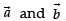we always have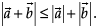Reason (R): The given inequality holds trivially when either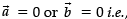in such a case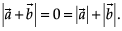Then consider

So, let us check it for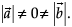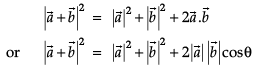For cos θ ≤ 1, we have :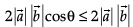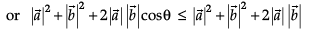or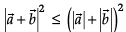or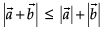## Mathematics (Maths) Class 12

206 videos|263 docs|139 tests
Information about Test: Vectors- Assertion & Reason Type Questions Page
In this test you can find the Exam questions for Test: Vectors- Assertion & Reason Type Questions solved & explained in the simplest way possible. Besides giving Questions and answers for Test: Vectors- Assertion & Reason Type Questions, EduRev gives you an ample number of Online tests for practice

## Mathematics (Maths) Class 12

206 videos|263 docs|139 tests